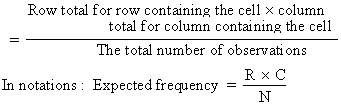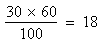Support the Monkey! Tell All your Friends and TeachersHome MonkeyNotes Printable Notes Digital Library Study Guides Study Smart Parents Tips College Planning Test Prep Fun Zone Help / FAQ How to Cite Request a New Title

8.21 Chi-Square

Tests like z-score, t, F are based on the assumption that the samples were drawn from normally distributed populations or more accurately that the sample means were normally distributed. As these tests require assumption about the type of population or parameters, these tests are known as 'parametric tests.'

There are many situations in which it is impossible to make any rigid assumption about the distribution of the population from which samples are drawn. This limitation led to search for non-parametric tests. Chi-square (Read as Ki - square) test of independence and goodness of fit is a prominent example of a non-parametric test. The chi-square ( c2 ) test can be used to evaluate a relationship between two nominal or ordinal variables.

c2 (chi-square) is measure of actual divergence of the observed and expected frequencies. In sampling studies we never expect that there will be a perfect coincidence between actual and observed frequencies and the question that we have to tackle is about the degree to which the difference between actual and observed frequencies can be ignored as arising due to fluctuations of sampling. if ;there is no difference between actual and observed frequencies then c2 = 0. If there is a difference, then c2 would be more than 0. But the difference may also be due to sample fluctuation and thus the value of c2 should be ignored in drawing the inference. Such values of c2 under different conditions are given in the form of tables and if the actual value is greater than the table value, it indicates that the difference is not solely due to sample fluctuation and that there is some other reason.

On the other hand, if the calculated c2 is less than the table value, it indicates that the difference may have arisen due to chance fluctuations and can be ignored. Thus c2-test enable us to find out the divergence between theory and fact or between expected and actual frequencies is significant or not.

If the calculated value of c2 is very small, compared to table value then expected frequencies are very little and the fit is good.

If the calculated value of c2 is very large as compared to table value then divergence between the expected and the observed frequencies is very big and the fit is poor.

We know that the degree of freedom r (df) is the number of independent constraints in a set of data.

Suppose there is a 2 ´ 2 association table and actual frequencies of the various classes are as follows :

 A a B AB aB 60 -22 -38 b Ab ab 40 -8 -32 30 70 100

Now the formula for calculating expected frequency of any class ( cell )Your browser does not support the IFRAME tag.

For example, if we have two attributes A and B that are independent then the expected frequency

of the class (cell) AB would beOnce the expected frequency of cell (AB) is decided the expected frequencies of remaining three classes are automatically fixed.

Thus   for class (aB) it would be 60 - 18 = 42

for class (Ab) it would be 30 - 18 = 12

for class (ab) it would be 70 - 42 = 28

This means that so far as 2 ´ 2 association ( contingency ). Table is concerned, there is 1 degree of freedom.

In such tables the degrees of freedom are given by a formula   n = (c - 1) (r - 1)

where c = Number of columns

r = Number of rows

Index

8.1 Population
8.2 Sample
8.3 Parameters and Statistic
8.4 Sampling Distribution
8.5 Sampling Error
8.6 Central Limit Theorem
8.7 Critical Region
8.8 Testing of Hypothesis
8.9 Errors in Tesitng of Hypothesis
8.10 Power o a Hypothesis Test
8.11 Sampling of Variables
8.12 Sampling of Attributes
8.13 Estimation
8.14 Testing the Difference Between Means
8.15 Test for Difference Between Proportions
8.16 Two Tailed and one Tailed Tests
8.17 Test of Significance for Small Samples
8.18 Students t-distribution
8.19 Distribution of 't' for Comparison of Two Samples Means Independent Samples
8.20 Testing Difference Between Mens of Two Samples Dependent Samples or Matched Paired Observations
8.21 Chi-Square
8.22 Sampling Theory of Correlation
8.23 Sampling Theory of Regression

Chapter 1Search: All Products Books Popular Music Classical Music Video DVD Toys & Games Electronics Software Tools & Hardware Outdoor Living Kitchen & Housewares Camera & Photo Cell Phones Keywords: